Context splitting and substructural terms
1st January 2014
12:00
liamoc.net

As someone who formalises many programming language semantics and type systems, I am perennially interested in elegant representations of terms and judgements in proof assistants. This is an area of research that has been thoroughly investigated and no universal solution has been found (although I get the feeling that Beluga is a step in the right direction), but in this post I will explore some representational tricks that can be used to elegantly encode calculi with substructural type systems such as linear types, and in the process share with you a couple of the formalisations I’ve been working with.

To start with, we’ll look at representing the simply typed lambda calculus of Church (1940):Representing these terms directly, using names, is a cumbersome burden in a proof assistant. Care must be taken to avoid capture in substitution, and, more importantly, α-equivalent terms are not definitionally equal.

Instead, a common technique is to represent the variable names as natural numbers which denote the number of binders that are in scope between the name and its corresponding binder. For example, the following image1 represents the lambda term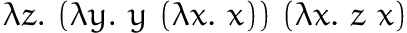:These indices, named after their inventor de Bruijn (1972), fit neatly into a dependently typed formalisation, with a little twist:

data Type : Set where
ι    : Type
_⇒_ : Type → Type → Type

data Term (n : ℕ) : Set where
var : Fin n → Term n
app : Term n → Term n → Term n
abs : Type → Term (suc n) → Term n


Instead of representing variable names as straight natural numbers, we parameterise terms by the number of available variables n, and then only allow available variables to be referenced inside the term by using the type of finite sets to represent variable names. This way, we tame some of the error-prone nature of de Bruijn indices by assigning different types to terms with differing numbers of available variables. Closed terms are represented as values of type Term 0. I first encountered this trick in McBride (2003), but I know not from whence it originated.

Representing the above typing rules in Agda is also nice, using length-indexed vectors of types to represent the type environment:

module STLC where
data _⊢_∶_ {n : ℕ} (Γ : Vec Type n) : Term n → Type → Set where

Var : ∀ {x}{τ}
→ Γ [ x ]= τ
-----------------
→ Γ ⊢ var x ∶ τ

App : ∀ {x y}{ρ τ}
→ Γ ⊢ x ∶ (ρ ⇒ τ)
→ Γ ⊢ y ∶ ρ
-------------------
→ Γ ⊢ app x y ∶ τ

Abs : ∀ {x}{ρ τ}
→ (τ ∷ Γ) ⊢ x ∶ ρ
-------------------------
→ Γ ⊢ abs τ x ∶ (τ ⇒ ρ)


This niceness all goes out the window, however, as soon as you start messing with the structure of contexts. In this post, I’ll be looking at the linear lambda calculus. For an approachable introduction to linear types, I highly recommend Wadler (1990), but I will give a brief introduction here.

In the typing rules above, we were treating our environment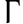as though it was a set of type assignments. An alternative presentation uses a list of assignments:Note that the application rule now splits the context, and the variable rule now disallows any additional judgements to be in the context. To recover our original type system, we must add some structural rules that make our context mimic a set:The first rule,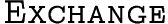, simply tells us that the order of judgements in the list doesn’t matter. The second rule,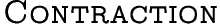, lets us use the knowledge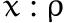in multiple places in the expression — in other words, it lets us use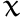more than once. Without this rule, we would have an affine type system. Note that affine type systems naturally disallow aliasing. The final rule,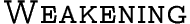lets us throw away knowledge thatwithout using it. Without this rule and therule, our type system is linear — all variables must be used exactly once.

Linear type systems have a number of interesting properties, discussed in Wadler (1990), but they pose a problem for our term representation above: The number of available variables is no longer syntax-directed! Instead, it is determined by a set of unruly typing rules. It seems as though the advantages of our de Bruijn representation above are now unattainable, however some niceness is retained by sticking with it — if we separate the notion of a variable that is in scope but unavailable (i.e. used elsewhere in the expression) from a variable that is simply out of scope, we can keep the term structure as-is and use a partial environment as our context:

Env : ℕ → Set
Env n = Vec (Maybe Type) n


The empty environment is more accurately a family of empty environments – environments where all in scope variables are unavailable:

ε : ∀ {n} → Env n
ε {zero}  = []
ε {suc n} = nothing ∷ ε


And the singleton environment, used in the Var rule, is defined similarly:

singleton : ∀ {n} → Fin n → Type → Env n
singleton zero    τ = just τ ∷ ε
singleton (suc n) τ = nothing ∷ singleton n τ


Then, the rules can be straightforwardly defined:

module Typing (_⇝_⊕_ : Maybe Type → Maybe Type → Maybe Type → Set) where
mutual
data _⊢_∶_ {n : ℕ} : Env n → Term n → Type → Set where

Var : ∀ {x}{τ}
-----------------------------
→ singleton x τ ⊢ var x ∶ τ

App : ∀ {x y}{ρ τ}{Γ Γ₁ Γ₂}
→ Γ ⇝ Γ₁ ⊞ Γ₂
→ Γ₁ ⊢ x ∶ (ρ ⇒ τ)
→ Γ₂ ⊢ y ∶ ρ
-------------------
→ Γ ⊢ app x y ∶ τ

Abs : ∀ {x}{ρ τ}{Γ}
→ (just τ ∷ Γ) ⊢ x ∶ ρ
-------------------------
→ Γ ⊢ abs τ x ∶ (τ ⇒ ρ)

Note that the application rule now explicitly splits the context, according to the following relation:
  data _⇝_⊞_ : ∀{n} → Env n → Env n → Env n → Set where
Empty : [] ⇝ [] ⊞ []
Cons  : ∀{n}{x x₁ x₂}{xs xs₁ xs₂ : Env n}
→ x  ⇝ x₁  ⊕ x₂
→ xs ⇝ xs₁ ⊞ xs₂
--------------------------------------
→ (x ∷ xs) ⇝ (x₁ ∷ xs₁) ⊞ (x₂ ∷ xs₂)

module Linear where
data _⇝_⊕_ : Maybe Type → Maybe Type → Maybe Type → Set where
Left  : ∀ {x} → x ⇝ x ⊕ nothing
Right : ∀ {x} → x ⇝ nothing ⊕ x

open Typing (_⇝_⊕_) public


This way, instead of expressing a split environment as a composition of two other environments, we represent context splitting as an explicit proof object, where the prover chooses which judgment goes to which expression.

We don’t need to encode the one remaining structural rule () as the order has already been canonicalised by the de Bruijn indices.

Now, suppose we wanted to express the normal simply-typed lambda calculus in this style2. How would we express the additional structural rules? Therule can be embedded within the context splitting relation:

module Relevant where
data _⇝_⊕_ : Maybe Type → Maybe Type → Maybe Type → Set where
Left  : ∀ {x} → x ⇝ x ⊕ nothing
Right : ∀ {x} → x ⇝ nothing ⊕ x
Contr : ∀ {x} → x ⇝ x ⊕ x

open Typing (_⇝_⊕_) public


This works because the only useful application of the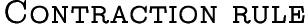is immediately before a context split. One might be tempted to try the same thing for:

module STLC′ where
data _⇝_⊕_ : Maybe Type → Maybe Type → Maybe Type → Set where
Left  : ∀ {x} → x ⇝ x ⊕ nothing
Right : ∀ {x} → x ⇝ nothing ⊕ x
Contr : ∀ {x} → x ⇝ x ⊕ x
Weak  : ∀ {x} → x ⇝ nothing ⊕ nothing

open Typing (_⇝_⊕_) public


This version makes obligations like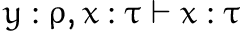unprovable, however. Instead, we should confine weakening to variable occurrences, just as we confine contraction to context splitting — another slight alteration must be made to the typing rules. The split relation remains the same:

module STLC″ where
data _⇝_⊕_ : Maybe Type → Maybe Type → Maybe Type → Set where
Left  : ∀ {x} → x ⇝ x ⊕ nothing
Right : ∀ {x} → x ⇝ nothing ⊕ x
Contr : ∀ {x} → x ⇝ x ⊕ x
data _⇝_⊞_ : ∀{n} → Env n → Env n → Env n → Set where
Empty : [] ⇝ [] ⊞ []
Cons  : ∀{n}{x x₁ x₂}{xs xs₁ xs₂ : Env n}
→ x  ⇝ x₁  ⊕ x₂
→ xs ⇝ xs₁ ⊞ xs₂
--------------------------------------
→ (x ∷ xs) ⇝ (x₁ ∷ xs₁) ⊞ (x₂ ∷ xs₂)
data _⊢_∶_ {n : ℕ} : Env n → Term n → Type → Set where


The typing rules differ, however, in that they now allow weakening in the above example, by relaxing the rule for variables3:

   Var : ∀ {x}{τ}{Γ}
→ Γ [ x ]= just τ
-------------------
→ Γ ⊢ var x ∶ τ

App : ∀ {x y}{ρ τ}{Γ Γ₁ Γ₂}
→ Γ ⇝ Γ₁ ⊞ Γ₂
→ Γ₁ ⊢ x ∶ (ρ ⇒ τ)
→ Γ₂ ⊢ y ∶ ρ
-------------------
→ Γ ⊢ app x y ∶ τ

Abs : ∀ {x}{ρ τ}{Γ}
→ (just τ ∷ Γ) ⊢ x ∶ ρ
-------------------------
→ Γ ⊢ abs τ x ∶ (τ ⇒ ρ)


Now, getting back to the split relation we defined earlier: it has a number of interesting properties. For starters, if one subcontext is ε, then the relation is simply equality:

 postulate split-eq  : ∀{n}{Γ₁ Γ₂ : Env n}
→ Γ₁ ⇝ Γ₂ ⊞ ε ⇔ Γ₁ ≡ Γ₂


The relation also is symmetric in the two subcontexts:

 postulate split-sym : ∀{n}{Γ Γ₁ Γ₂ : Env n}
→ Γ ⇝ Γ₂ ⊞ Γ₁
→ Γ ⇝ Γ₁ ⊞ Γ₂


The relation has a number of properties that look like transitivity. One is the following:

 postulate split-trans-genL : ∀{n}{Γ₁ Γ₂ Γ₃ Γ₁′ Γ₂′ : Env n}
→ Γ₁ ⇝ Γ₂ ⊞ Γ₁′
→ Γ₂ ⇝ Γ₃ ⊞ Γ₂′
→ ∃ (λ Δ → Γ₁ ⇝ Γ₃  ⊞ Δ
× Δ  ⇝ Γ₂′ ⊞ Γ₁′)


This theorem is captured by the below diagram: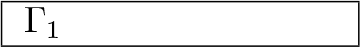That is, if a context is split, and then split again, then you could split the context from the other direction and still end up with the same results.

An alternative, rightward-skewed version of the lemma exists as well, as a direct consequence of split-sym:

 postulate split-trans-genR : ∀{n}{Γ₁ Γ₂ Γ₃ Γ₁′ Γ₂′ : Env n}
→ Γ₁ ⇝ Γ₁′ ⊞ Γ₂
→ Γ₂ ⇝ Γ₂′ ⊞ Γ₃
→ ∃ (λ Δ → Γ₁ ⇝ Δ ⊞ Γ₃
× Δ  ⇝ Γ₁′ ⊞ Γ₂′)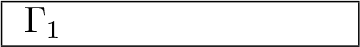Another transitivity-ish property works simultaneously over both subcontexts:
 postulate split-trans-simul : ∀{n}{Γ Γ₁ Γ₂ Γ₁₁ Γ₁₂ Γ₂₁ Γ₂₂ : Env n}
→ Γ ⇝ Γ₁ ⊞ Γ₂
→ Γ₁ ⇝ Γ₁₁ ⊞ Γ₁₂
→ Γ₂ ⇝ Γ₂₁ ⊞ Γ₂₂
→ ∃ (λ Γ□₁ → Γ□₁ ⇝ Γ₁₁ ⊞ Γ₂₁)
× ∃ (λ Γ□₂ → Γ□₂ ⇝ Γ₁₂ ⊞ Γ₂₂)


This theorem can be visualised by the following diagram, which requires an additional dimension to adequately convey the intuition: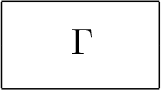In other words, if a context is split and then both subcontexts are split again, the original context can be split into different subcontexts and split again, and the same subsubcontexts will be produced.

I find this relation rather curious. If anyone has seen a relation with this structure before, or knows an algebraic abstraction that applies to it, I’d be very interested to hear your thoughts.

My preliminary work using a formalisation of this style shows that using this relation to specify a type system for a language which includes linear types is quite comfortable, in both Isabelle and Agda, although in Agda the proofs are quite a bit more tedious. If anyone knows of other approaches to this problem, though, I would definitely be interested to see them.

Church, A. (1940). A Formulation of the Simple Theory of Types. The Journal of Symbolic Logic, 5(2), 56–68.
de Bruijn, N. G. (1972). Lambda Calculus Notation with Nameless Dummies: a Tool for Automatic Formula Manipulation with Application to the Church-Rosser Theorem. Indagationes Mathematicae (Elsevier), 34, 381–392.
McBride, C. (2003). First-Order Unification by Structural Recursion. Journal of Functional Programming, 13(6), 1061–1075.
Wadler, P. (1990). Linear types can change the world! In M. Broy & C. Jones, eds., IFIP TC 2 working conference on programming concepts and methods, sea of galilee, israel, North Holland Publishing, pp. 347–359.

1. Shamelessly stolen from Wikipedia.↩︎

2. A more realistic scenario is that we want to mix linear and non-linear types in the same type system, but this raises a host of issues with closure capture that I don’t want to discuss in detail here. See Wadler (1990) for details.↩︎

3. Generalising this to a mixed linear/non-linear type system, one would require a relation Γ strongerThan Γ′ that allows weakening only for nonlinear variables, and then just have the typing rule Γ strongerThan singleton x τ → Γ ⊢ var x ∶ τ for variable occurrences.↩︎

agda term-representation verification types semantics linear-types cogent